# chapter 2 revised.pptx

May. 31, 2023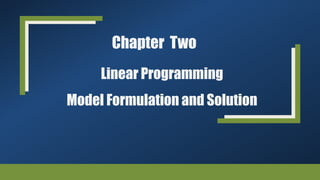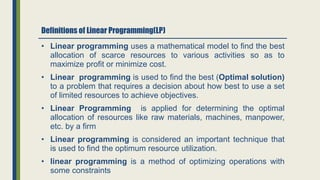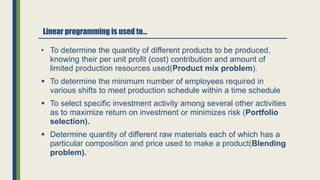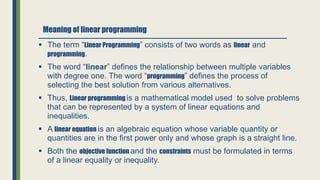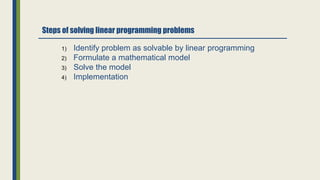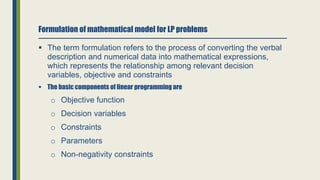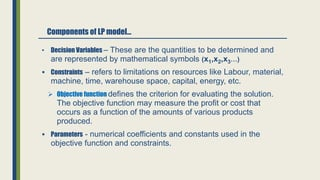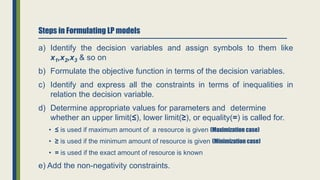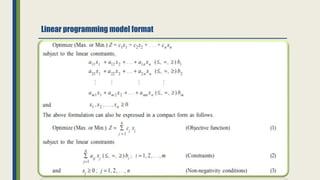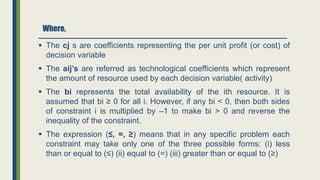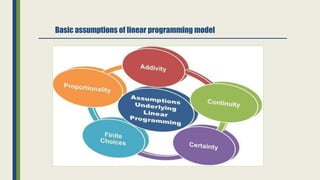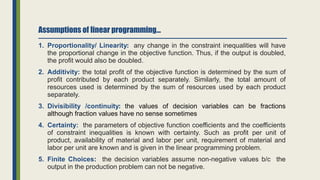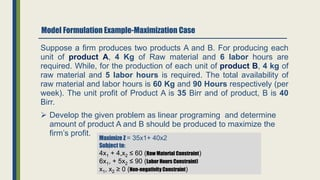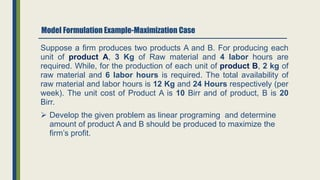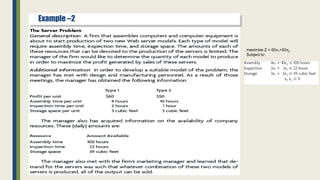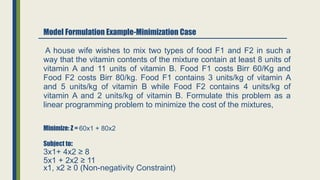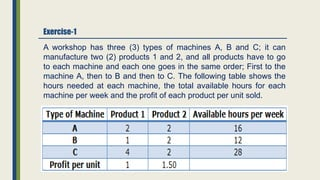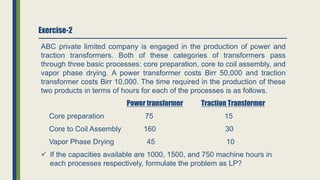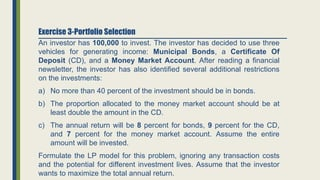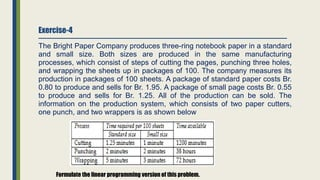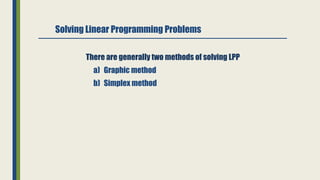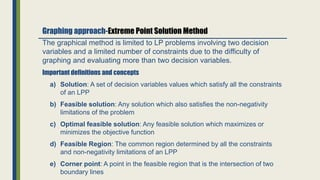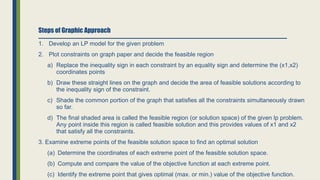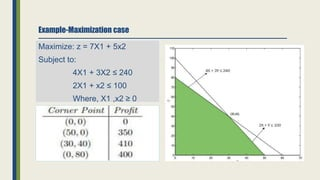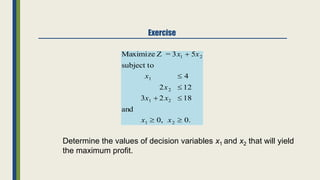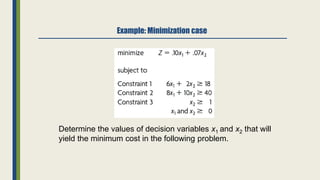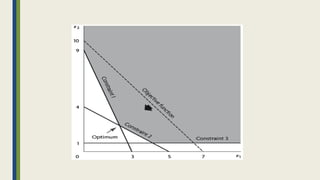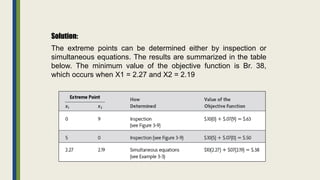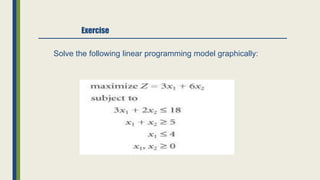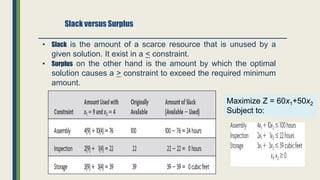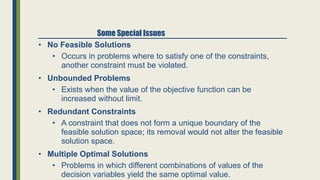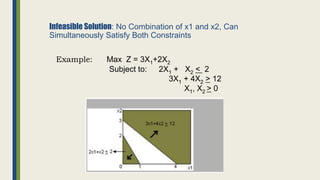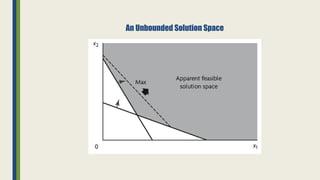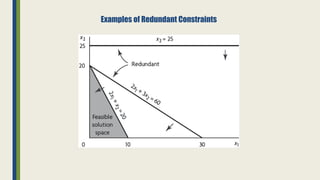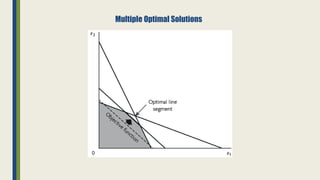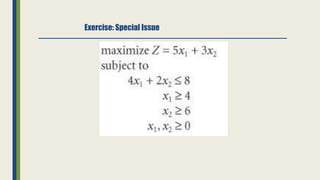1 of 36

### chapter 2 revised.pptx

• 1. Chapter Two Linear Programming Model Formulation and Solution
• 2. Definitions of Linear Programming(LP) • Linear programming uses a mathematical model to find the best allocation of scarce resources to various activities so as to maximize profit or minimize cost. • Linear programming is used to find the best (Optimal solution) to a problem that requires a decision about how best to use a set of limited resources to achieve objectives. • Linear Programming is applied for determining the optimal allocation of resources like raw materials, machines, manpower, etc. by a firm • Linear programming is considered an important technique that is used to find the optimum resource utilization. • linear programming is a method of optimizing operations with some constraints
• 3. Linear programming is used to… • To determine the quantity of different products to be produced, knowing their per unit profit (cost) contribution and amount of limited production resources used(Product mix problem).  To determine the minimum number of employees required in various shifts to meet production schedule within a time schedule  To select specific investment activity among several other activities as to maximize return on investment or minimizes risk (Portfolio selection).  Determine quantity of different raw materials each of which has a particular composition and price used to make a product(Blending problem).
• 4. Meaning of linear programming  The term “Linear Programming” consists of two words as linear and programming.  The word “linear” defines the relationship between multiple variables with degree one. The word “programming” defines the process of selecting the best solution from various alternatives.  Thus, Linear programming is a mathematical model used to solve problems that can be represented by a system of linear equations and inequalities.  A linear equation is an algebraic equation whose variable quantity or quantities are in the first power only and whose graph is a straight line.  Both the objective function and the constraints must be formulated in terms of a linear equality or inequality.
• 5. 1) Identify problem as solvable by linear programming 2) Formulate a mathematical model 3) Solve the model 4) Implementation Steps of solving linear programming problems
• 6. Formulation of mathematical model for LP problems  The term formulation refers to the process of converting the verbal description and numerical data into mathematical expressions, which represents the relationship among relevant decision variables, objective and constraints  The basic components of linear programming are o Objective function o Decision variables o Constraints o Parameters o Non-negativity constraints
• 7. Components of LP model… • Decision Variables – These are the quantities to be determined and are represented by mathematical symbols (x1,x2,x3…)  Constraints – refers to limitations on resources like Labour, material, machine, time, warehouse space, capital, energy, etc.  Objective function defines the criterion for evaluating the solution. The objective function may measure the profit or cost that occurs as a function of the amounts of various products produced.  Parameters - numerical coefficients and constants used in the objective function and constraints.
• 8. Steps in Formulating LP models a) Identify the decision variables and assign symbols to them like x1,x2,x3 & so on b) Formulate the objective function in terms of the decision variables. c) Identify and express all the constraints in terms of inequalities in relation the decision variable. d) Determine appropriate values for parameters and determine whether an upper limit(≤), lower limit(≥), or equality(=) is called for. • ≤ is used if maximum amount of a resource is given (Maximization case) • ≥ is used if the minimum amount of resource is given (Minimization case) • = is used if the exact amount of resource is known e) Add the non-negativity constraints.
• 10. Where,  The cj s are coefficients representing the per unit profit (or cost) of decision variable  The aij’s are referred as technological coefficients which represent the amount of resource used by each decision variable( activity)  The bi represents the total availability of the ith resource. It is assumed that bi ≥ 0 for all i. However, if any bi < 0, then both sides of constraint i is multiplied by –1 to make bi > 0 and reverse the inequality of the constraint.  The expression (≤, =, ≥) means that in any specific problem each constraint may take only one of the three possible forms: (i) less than or equal to (≤) (ii) equal to (=) (iii) greater than or equal to (≥)
• 11. Basic assumptions of linear programming model
• 12. Assumptions of linear programming… 1. Proportionality/ Linearity: any change in the constraint inequalities will have the proportional change in the objective function. Thus, if the output is doubled, the profit would also be doubled. 2. Additivity: the total profit of the objective function is determined by the sum of profit contributed by each product separately. Similarly, the total amount of resources used is determined by the sum of resources used by each product separately. 3. Divisibility /continuity: the values of decision variables can be fractions although fraction values have no sense sometimes 4. Certainty: the parameters of objective function coefficients and the coefficients of constraint inequalities is known with certainty. Such as profit per unit of product, availability of material and labor per unit, requirement of material and labor per unit are known and is given in the linear programming problem. 5. Finite Choices: the decision variables assume non-negative values b/c the output in the production problem can not be negative.
• 13. Model Formulation Example-Maximization Case Suppose a firm produces two products A and B. For producing each unit of product A, 4 Kg of Raw material and 6 labor hours are required. While, for the production of each unit of product B, 4 kg of raw material and 5 labor hours is required. The total availability of raw material and labor hours is 60 Kg and 90 Hours respectively (per week). The unit profit of Product A is 35 Birr and of product, B is 40 Birr.  Develop the given problem as linear programing and determine amount of product A and B should be produced to maximize the firm’s profit. Maximize Z = 35x1+ 40x2 Subject to: 4x1 + 4,x2 ≤ 60 (Raw Material Constraint) 6x1, + 5x2 ≤ 90 (Labor Hours Constraint) x1, x2 ≥ 0 (Non-negativity Constraint)
• 14. Model Formulation Example-Maximization Case Suppose a firm produces two products A and B. For producing each unit of product A, 3 Kg of Raw material and 4 labor hours are required. While, for the production of each unit of product B, 2 kg of raw material and 6 labor hours is required. The total availability of raw material and labor hours is 12 Kg and 24 Hours respectively (per week). The unit cost of Product A is 10 Birr and of product, B is 20 Birr.  Develop the given problem as linear programing and determine amount of product A and B should be produced to maximize the firm’s profit.
• 15. Example –2 maximize Z = 60x1+50x2 Subject to:
• 16. Model Formulation Example-Minimization Case Minimize: Z = 60x1 + 80x2 Subject to: 3x1+ 4x2 ≥ 8 5x1 + 2x2 ≥ 11 A house wife wishes to mix two types of food F1 and F2 in such a way that the vitamin contents of the mixture contain at least 8 units of vitamin A and 11 units of vitamin B. Food F1 costs Birr 60/Kg and Food F2 costs Birr 80/kg. Food F1 contains 3 units/kg of vitamin A and 5 units/kg of vitamin B while Food F2 contains 4 units/kg of vitamin A and 2 units/kg of vitamin B. Formulate this problem as a linear programming problem to minimize the cost of the mixtures, x1, x2 ≥ 0 (Non-negativity Constraint)
• 17. A workshop has three (3) types of machines A, B and C; it can manufacture two (2) products 1 and 2, and all products have to go to each machine and each one goes in the same order; First to the machine A, then to B and then to C. The following table shows the hours needed at each machine, the total available hours for each machine per week and the profit of each product per unit sold. Exercise-1
• 18. Exercise-2 ABC private limited company is engaged in the production of power and traction transformers. Both of these categories of transformers pass through three basic processes: core preparation, core to coil assembly, and vapor phase drying. A power transformer costs Birr 50,000 and traction transformer costs Birr 10,000. The time required in the production of these two products in terms of hours for each of the processes is as follows. Power transformer Traction Transformer Core preparation 75 15 Core to Coil Assembly 160 30 Vapor Phase Drying 45 10  If the capacities available are 1000, 1500, and 750 machine hours in each processes respectively, formulate the problem as LP?
• 19. An investor has 100,000 to invest. The investor has decided to use three vehicles for generating income: Municipal Bonds, a Certificate Of Deposit (CD), and a Money Market Account. After reading a financial newsletter, the investor has also identified several additional restrictions on the investments: a) No more than 40 percent of the investment should be in bonds. b) The proportion allocated to the money market account should be at least double the amount in the CD. c) The annual return will be 8 percent for bonds, 9 percent for the CD, and 7 percent for the money market account. Assume the entire amount will be invested. Formulate the LP model for this problem, ignoring any transaction costs and the potential for different investment lives. Assume that the investor wants to maximize the total annual return. Exercise 3-Portfolio Selection
• 20. Exercise-4 The Bright Paper Company produces three-ring notebook paper in a standard and small size. Both sizes are produced in the same manufacturing processes, which consist of steps of cutting the pages, punching three holes, and wrapping the sheets up in packages of 100. The company measures its production in packages of 100 sheets. A package of standard paper costs Br. 0.80 to produce and sells for Br. 1.95. A package of small page costs Br. 0.55 to produce and sells for Br. 1.25. All of the production can be sold. The information on the production system, which consists of two paper cutters, one punch, and two wrappers is as shown below Formulate the linear programming version of this problem.
• 21. Solving Linear Programming Problems There are generally two methods of solving LPP a) Graphic method b) Simplex method
• 22. The graphical method is limited to LP problems involving two decision variables and a limited number of constraints due to the difficulty of graphing and evaluating more than two decision variables. Important definitions and concepts a) Solution: A set of decision variables values which satisfy all the constraints of an LPP b) Feasible solution: Any solution which also satisfies the non-negativity limitations of the problem c) Optimal feasible solution: Any feasible solution which maximizes or minimizes the objective function d) Feasible Region: The common region determined by all the constraints and non-negativity limitations of an LPP e) Corner point: A point in the feasible region that is the intersection of two boundary lines Graphing approach-Extreme Point Solution Method
• 23. Steps of Graphic Approach 1. Develop an LP model for the given problem 2. Plot constraints on graph paper and decide the feasible region a) Replace the inequality sign in each constraint by an equality sign and determine the (x1,x2) coordinates points b) Draw these straight lines on the graph and decide the area of feasible solutions according to the inequality sign of the constraint. c) Shade the common portion of the graph that satisfies all the constraints simultaneously drawn so far. d) The final shaded area is called the feasible region (or solution space) of the given lp problem. Any point inside this region is called feasible solution and this provides values of x1 and x2 that satisfy all the constraints. 3. Examine extreme points of the feasible solution space to find an optimal solution (a) Determine the coordinates of each extreme point of the feasible solution space. (b) Compute and compare the value of the objective function at each extreme point. (c) Identify the extreme point that gives optimal (max. or min.) value of the objective function.
• 24. Example-Maximization case Maximize: z = 7X1 + 5x2 Subject to: 4X1 + 3X2 ≤ 240 2X1 + x2 ≤ 100 Where, X1 ,x2 ≥ 0
• 25. Exercise Maximize Z = 3x1  5x2 subject to x1  4 2x2  12 3x1  2x2  18 and x1  0, x2  0. Determine the values of decision variables x1 and x2 that will yield the maximum profit.
• 26. Example: Minimization case Determine the values of decision variables x1 and x2 that will yield the minimum cost in the following problem.
• 28. Solution: The extreme points can be determined either by inspection or simultaneous equations. The results are summarized in the table below. The minimum value of the objective function is Br. 38, which occurs when X1 = 2.27 and X2 = 2.19
• 29. Exercise Solve the following linear programming model graphically:
• 30. Slack versus Surplus • Slack is the amount of a scarce resource that is unused by a given solution. It exist in a < constraint. • Surplus on the other hand is the amount by which the optimal solution causes a > constraint to exceed the required minimum amount. Maximize Z = 60x1+50x2 Subject to:
• 31. • No Feasible Solutions • Occurs in problems where to satisfy one of the constraints, another constraint must be violated. • Unbounded Problems • Exists when the value of the objective function can be increased without limit. • Redundant Constraints • A constraint that does not form a unique boundary of the feasible solution space; its removal would not alter the feasible solution space. • Multiple Optimal Solutions • Problems in which different combinations of values of the decision variables yield the same optimal value. Some Special Issues
• 32. Infeasible Solution: No Combination of x1 and x2, Can Simultaneously Satisfy Both Constraints Example: Max Z = 3X1+2X2 Subject to: 2X1 + X2 < 2 3X1 + 4X2 > 12 X1, X2 > 0
• 34. Examples of Redundant Constraints